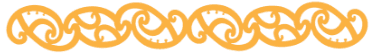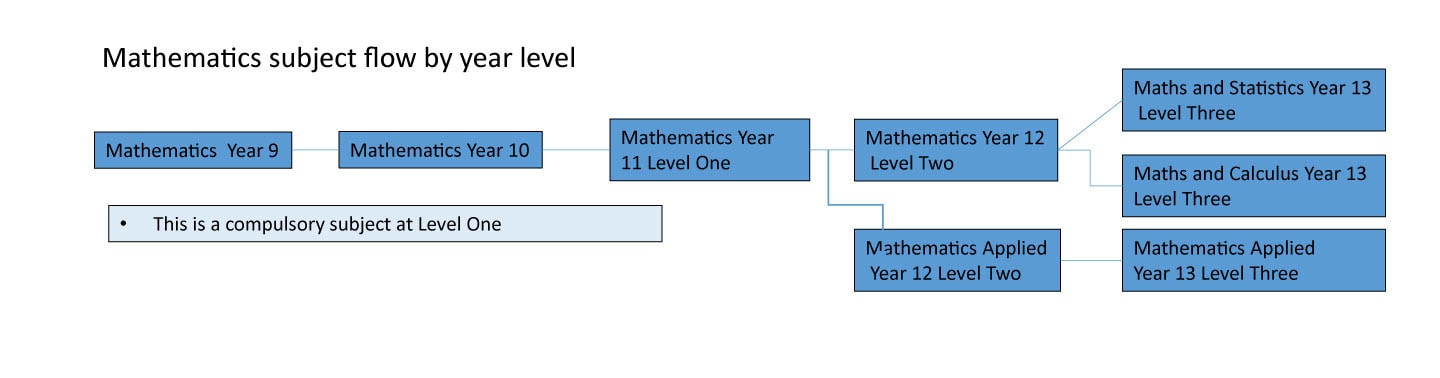# Mathematics# Mathematics

As abstract as that may seem, math is, at its core, a quest for absolutes, definitive solutions and answers. Math can teach us how to look longer and harder for solutions – a skill applicable to any career and life in general. Whether students like mathematics or not or math is around us all the time.

Many professions, such as engineering, medicine, physics, nurses, computer science and actuarial science, require math proficiency. Most fields benefit from the analytical and problem-solving skills students learn in mathematics. Anyone entering a science, technology, engineering and mathematics (STEM) career is expected to have understand basic and advanced math concepts.

Mathematics is done by all students through to Level 1. At Level 2 they have the option of Mathematics or Mathematics Applied. At Level Three they have the option of Maths and Statistics, Maths and Calculus or Maths Applied.